Luo, P., Cao, C., Liang, Y., Ma, X., Xin, C., Jiao, Z., Cao, J., and Zhang, J. (2013). "Kinetic study of the acetylation of cotton linter pulp," BioRes. 8(2), 2708-2718.

#### Abstract

The kinetics of the reaction of cotton linter pulp with acetic anhydride catalyzed by sulfuric acid at temperatures ranging from 30 to 45 °C have been investigated. A kinetic model considering the dissolution of the sufficiently sulfated cellulose chains on the surface of the solid fibers, and the subsequent reactions in the liquid phase was proposed for the acetylation process. The kinetic parameters were regressed from the measured degree of substitution using a nonlinear regression method. With the optimum values of the rate constants, the proposed model can predict the acetylation process very well. The chemical activation energy of the reactions of the cellulose hydroxyl groups with acetic anhydride was estimated as 39.6 kJ/mol. The dissolution rate of the sulfated chains can be represented by the equation r/r0=1-kt. A similar equation, the Arrhenius expression, k=Aexp(-E/RT) was used to predict the dissolution rate constants. The corresponding activation energy for the dissolution was evaluated as 19.6 kJ/mol.

Kinetic Study of the Acetylation of Cotton Linter Pulp

Peicheng Luo,a,* Cuicui Cao,a Yinchun Liang,b,* Xiaolong Ma,b Chuanxian Xin,Zhen Jiao,a Jianhua Cao,b and Jie Zhang b

The kinetics of the reaction of cotton linter pulp with acetic anhydride catalyzed by sulfuric acid at temperatures ranging from 30 to 45 °C have been investigated. A kinetic model considering the dissolution of the sufficiently sulfated cellulose chains on the surface of the solid fibers, and the subsequent reactions in the liquid phase was proposed for the acetylation process. The kinetic parameters were regressed from the measured degree of substitution using a nonlinear regression method. With the optimum values of the rate constants, the proposed model can predict the acetylation process very well. The chemical activation energy of the reactions of the cellulose hydroxyl groups with acetic anhydride was estimated as 39.6 kJ/mol. The dissolution rate of the sulfated chains can be represented by the equation r/r0=1-kt. A similar equation, the Arrhenius expression, k=Aexp(-E/RT) was used to predict the dissolution rate constants. The corresponding activation energy for the dissolution was evaluated as 19.6 kJ/mol.

Keywords: Acetylation; Cotton linter; Cellulose acetate; Kinetics; Modeling; Dissolution

Contact information: a: School of Chemistry and Chemical Engineering, Southeast University, Nanjing 211189, China; b: Nantong Cellulose Fibers Co., Ltd., Nantong 226008, China;

* Corresponding authors: luopeicheng@seu.edu.cn; liangyinchun@gmail.com

INTRODUCTION

The application of cellulose acetate in cigarette filters, as well as in the fiber and textile industries has motivated a wide range of investigations for the acetylation process since the 1940s (Malm et al.1946). There has, however, been relatively little published work directed towards the kinetics of the process for proprietary reasons (Dubey et al. 2006). In a typical commercial process, strong acids, e.g.sulfuric acid, are always used as the catalyst. Since cellulose exists in the solid phase, it creates a multiphase reaction system, which also hinders the kinetic investigation of the process. By using simplified assumptions, Frith (1963) interpreted the results in terms of a non-diffusion controlled reaction and found that a first-order kinetic treatment was valid for the acetylation process catalyzed by perchloric acid. On the contrary, Sen and Ramaswamy (1957) concluded that the chemical reaction was so rapid that the diffusion alone was the rate-controlling process. Although diffusion was considered, they still estimated the kinetic parameters qualitatively and got the apparent activation energy.

In a later study, the acetylation process was investigated from ultrastructural aspects, including morphology observations and crystal structure analysis by microscopic techniques, e.g. scanning electron microscopy (SEM), transmission electron microscopy (TEM), and X-ray diffraction (XRD) experiments (Frisoni et al. 2001; Sassi and Chanzy 1995; Tang et al. 1997; Yang et al. 2008). Sassi and Chanzy (1995) distinguished two acetylation mechanisms, the ‘fibrous process’ and the ‘homogeneous process’, depending on the presence or absence of a non-swelling solvent. For a ‘fibrous process’ mechanism, solvents such as toluene, benzene, or amyl acetate are added to the reaction medium, and cellulose remains insoluble in the reaction medium, i.e. there is a direct conversion of cellulose into solid cellulose triacetate (CTA) without change in the morphology of the fibers. The application of ‘fibrous acetylation process’ in the field of wood modification has provided an incentive for kinetic investigations on this process. For instance, Hill et al. (1998) studied the kinetics of the reaction of acetic anhydride with wood block in pyridine. In their study, the diffusion of the reagent into the wood matrix was considered. Minato (2004) also proposed a modified first-order rate equation in which the effect of diffusion was taken into consideration.

For a ‘homogeneous process’ mechanism, a more common solution, e.g. acetic acid, is used and CTA is solubilized in the reaction medium. In this case, the cellulose fibers undergo substantial morphological changes. By using TEM and XRD observations of the process, Sassi and Chanzy (1995) proposed a mechanism model to describe the ‘homogeneous process’, i.e. when the cellulose chains on the surface of the solid fibers become acetylated, they will become soluble and leave the crystal into the reaction medium. Then, the new surface will be exposed for the subsequent reaction. Therefore, the acetylation rate will be determined by both the dissolution of the reacted chains and the intrinsic reaction rate of hydroxyl groups with acetic anhydride.

The objective of this study was to investigate the kinetic aspects of the ‘homogeneous acetylation process’ based on the assumptions made by Sassi and Chanzy (1995). Cotton linter was selected as the cellulose samples for its potential alternative to wood pulp for the commercial acetylation processes. The dissolution of the sulfated layers or chains was incorporated into the mathematical model.

EXPERIMENTAL

Materials

Cotton linter pulp (as seen in Fig. 1a) was supplied by Nantong Linan Industrial & Trading Co., Ltd. The moisture content of the pulp was about 7%. The pulp was then shredded in an industrial shredder to make it fluffy (as seen in Fig. 1b). SEM observation showed that the fibers had a rather smooth surface (see Fig. 1c). Two-hundred measurements of the fibers with polarizing microscope (example shown in Fig. 1d) were analyzed, yielding an average value of 19.0 μm in diameter and 1495 μm in length.

Reagent-quality acetic acid (99.5%), acetic anhydride (98.5%), and sulfuric acid (98%) were supplied by Sinopharm Chemical Reagent Co., Ltd.

Acetylation

A typical acetylation procedure was adopted in the present study (Steinmeier 2004). Before each acetylation process, the cotton linter pulp was dried in a vacuum for 24 h. A mixture (Mixture A) of 2 grams dry cotton linter pulp and 80 grams acetic acid was pre-treated by shaking for 1 h. Twenty grams mixed acid of acetic acid (57.3 wt%) and acetic anhydride (42.7wt%), and 0.1 grams sulfuric acid (98%) were mixed and added to a 250-mL round bottom flask, which was set in a water bath to regulate the temperature. According to the operation conditions of the present commercial acetylation process, the temperature was fixed at 30, 35, 40, and 45 °C (±0.2), respectively. When the system reached the desired temperature, Mixture A (pre-heated to the desired temperature) was then added to the flask and the acetylation process was carried out for 30 to 120 min at the desired temperature. During the whole process, an impeller agitator was used to make the cotton linter pulp well dispersed and the liquid reaction medium homogeneous. The speed of the agitator was fixed at 600 rpm. Samples of the reaction mixture were taken at intervals and immediately added to a mixture of aqueous solution of magnesium acetate (5 wt%) and acetic acid (20 wt%) to quench the reaction. These samples were then precipitated in 20% aqueous solution of sodium chloride, washed with water repeatedly to neutrality, and dried in a vacuum at room temperature.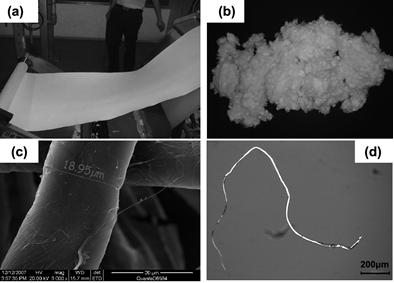Fig. 1. Morphology pictures of cotton linter pulp (a) before and (b) after shredding treatment, and microscopic observations of the fibers by (c) scanning electron microscope and (d) polarization microscope

Perpropionylation

Samples (0.3 g) after the acetylation procedure were dissolved in 5 mL of dry pyridine. The solution was treated with 5 mL of propionic anhydride and stirred at 80 °C for 24 h. After cooling down, the product was precipitated into 200 mL of dry methanol. The solid was filtered and washed three times with methanol. Finally, the perpropionylated cellulose acetate was dried in a vacuum at 60 °C.

Degree of Substitution (DS) Determination by 1H-NMR

For the structural analysis and the DS determination, Goodlett et al. (1971) made the first attempt to analyze the structure of cellulose acetate using 1H-NMR. It is found that cellulose triacetate yields a very well-resolved, simple 1H-NMR spectrum, while partially substituted cellulose acetate (CA) samples show very complex spectra resulting from the un-, mono-, di-, and tri-substituted units with different combinations of the functionalized sites. Heinze and Lieber (2004) proposed an improved method to measure the DS by the complete propionylation of the remaining hydroxyl groups of CA. These spectra confirm the acetylation as well and can be used to analyze the distribution of the ester moieties within the repeating unit. 1H-NMR spectra were measured with a Bruker AV 300 spectrometer by using a standard 5 mm probe at room temperature. The acetylated cellulose samples (DS<3) were measured after perpropionylation in CDCl3 to determine the DS.

RESULTS AND DISCUSSION

Process Description

As stated above, the dissolution of the reacted chains from the surface of the solid fibers, the diffusion of the reagents in the bulk of the liquid phase, the diffusion of the reagents, e.g. sulfuric acid, from liquid phase to the surface of the solid cellulose, and the intrinsic reactivity of the hydroxyl groups should be taken into account for the kinetic study of the acetylation process. Figure 2 represents a schematic mechanism of the solid-liquid acetylation process. In the mechanism model proposed by Sassi and Chanzy (1995), when the chains of cellulose on the surface are sufficiently acetylated, they will become soluble and leave the solid surface of fibers into the liquid phase, thus leading to a reduction in the diameter of the crystalline cellulose while their longitudinal dimension stays either constant or is only slightly reduced. Indeed, compared to the sulfation, the acetylation reaction is relatively slow (Araki 1952; Malm et al. 1946). Moreover, cellulose sulfate ester has greater solubility in the reaction medium than cellulose acetate (Malm et al. 1961), i.e. it is the sulfate reaction that helps to dissolve the solid pulp by substituting one of its hydroxyl groups by the sulfate group. This reaction occurs exclusively at the primary hydroxyl group of cellulose (Dubey et al. 2006). Thus, the acetylation process involves two steps: the dissolution of the sulfated chains on the surface, and the subsequent reactions in the liquid phase including the acetylation of hydroxyl groups and the displacement of sulfate with the acetyl group by acetic anhydride. It has been noted that the acetylation rate of the primary hydroxyl groups is higher than that of the secondary groups (Malm et al. 1961). In this work, to simplify the model for this complicated process, it is assumed that these hydroxyl groups have the same reactivity.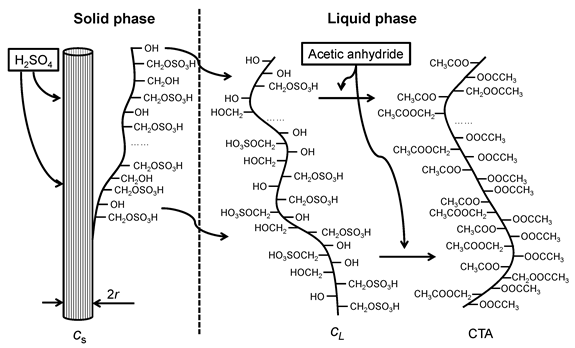Fig. 2. Schematic diagram of the acetylation process involving the dissolution of the reacted chains and the acetylation reactions of the hydroxyl groups

To eliminate the effect of the diffusion of the reagents, a very large excess of the solvent, acetic acid, as well as strong stirring of the mixture, was used in this study. Therefore, only the dissolution of the sulfated chains and the intrinsic reactions of hydroxyl groups with acetic anhydride were considered in the mathematical model.

Kinetic Model

When sufficient primary hydroxyl groups have been sulfated, the chains come to a critical state, i.e.further sulfation of the hydroxyl groups will make the chains soluble and they will enter into the liquid phase. The number of the critical cellulose chains on the surface has a proportional relationship with the surface area of the cellulose fiber, thus: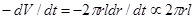(1)

where V is the volume of the cellulose fiber, r the radius of the fiber, l the length of the fiber, and t is time. When the concentration of sulfuric acid in the system is constant, Eq. 1 can be rewritten as,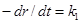(2)

where k1 is defined as the dissolution rate constant of the critical cellulose chains. It represents how fast the cellulose chains enter into the liquid reaction medium after they have been sufficiently sulfated. Obviously, k1 will be determined by the natural characteristics of the cellulose crystal and the concentration of sulfuric acid. When k1 is constant, Eq. 2 can be solved with the boundary condition of the initial radius of the fiber, r0,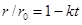(3)

where, k is a radius-independent dissolution rate constant, and k=k1/r0. It is assumed that there are nidentical thin cylindrical cellulose fibers. At the reaction time of t, the concentration of unreacted hydroxyl groups on the solid cellulose, cs, can be written on the basis of the total volume of the reaction mixture, Vr.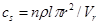(4)

where, ρ is the density of hydroxyl groups of solid cellulose fibers, kmol/m3. The initial concentration of hydroxyl groups, cs0, is: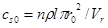(5)

When the critical sulfated chains enter into the liquid phase, hydroxyl groups will react with acetic anhydride. It is known that this reaction follows the second order rate laws (Hill et al. 1998). When the acetic anhydride is present in large excess, the reaction can be regarded as a pseudo first-order reaction. Thus,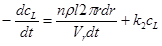(6)

where, cL is the concentration of unreacted hydroxyl groups in the liquid reaction medium and k2 is the pseudo first-order reaction constants. It is noted that Eq. 6 is only valid before all the solid cellulose fibers are dissolved into the liquid phase. Combined with Eqs. 3, 4, and 5, Eq. 6 can be resolved as follows: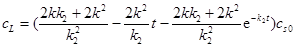(7)

The degree of substitution, DS, can be represented by Eq. 8 when t ≤ 1/k.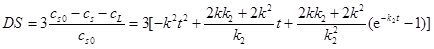(8)

When all the solid cellulose fibers have been dissolved into the liquid phase, i.e. t ≥ 1/k, the reaction rate only depends on the concentration of the unreacted hydroxyl groups, thus: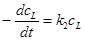(9)

The corresponding DS can be derived as Eq. 10.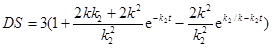(10)

DS results by 1H-NMR

The 1H-NMR spectrum after complete propionylation of the remaining hydroxyl groups of CA samples is shown in Fig. 3. The spectrum shows the characteristic signals of the anhydroglucose unit (AGU) at δ=3.5-5.1 ppm, the methylene proton of the propionate at δ=2.4 ppm, the methyl protons of acetate groups at δ=2.2, 1.9, 1.8 ppm (which are assigned to the acetyl moieties attached to position 6, 2, and 3, respectively), and the methyl proton of the propionate at δ=1.2 ppm. These NMR data are in accordance with those reported in the literature (Schlufter et al. 2006). As expected, the peaks of propionate and acetate are completely separate. Thus, the DS values are calculated from the ratio of the spectral integrals of acetate-CH3 and propionate-CH2 (or propionate-CH3, as seen in Fig. 3).

The DS results at different reaction temperature are shown in Fig. 4. A standard titration method (Steinmeier 2004) for the determination of DS was also used to evaluate the accuracy of NMR method. It is noted that the titration method is only appropriate for the case with high DS values. The comparison shows that the discrepancy of DS values between the two methods was below 5%.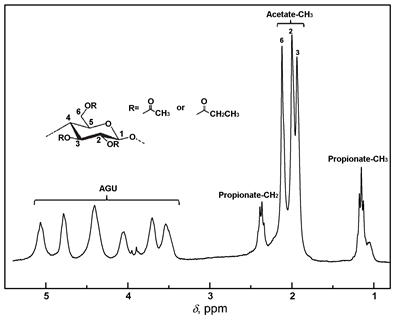Fig. 3. 1H-NMR spectrum of the perpropionylated sample in CDCl3 (acetylation reaction temperature, 40 °C; reaction time, 45 min)

Estimation of the Kinetic Parameters

Figure 4 shows the relation between the degree of substitution, DS, and the reaction time, t, at different reaction temperatures. It is noted that the lower limit of the reaction temperature is 30 °C. The reaction is much slower when the temperature is below 30 °C. Moreover, the upper limit of the reaction temperature is 45 °C, at which the reaction is very fast. One can see that DS approaches 2.66 after 45 min at 45 °C. In addition, temperatures ranging from 30 °C to 45 °C have always been used for commercial acetylation processes (Dubey et al. 2006). As discussed above, the DS values of the process can be calculated from Eqs. 8 and 10. A commercial computer program embedded in Matlab® for nonlinear regression based on the known damped least-squares method was used to estimate the radius-independent dissolution rate constants, k, and pseudo first-order reaction rate constants, k2. Lines in Fig. 4 show the regression curves using the proposed kinetic model, with R2=1.97E-3, 4.49E-4, 1.88E-4, and 1.10E-3 at 30 °C, 35 °C, 40 °C, and 45 °C, respectively. It can be seen that the nonlinear regression profiles predicted DS values very well nearly at all temperatures.

By the nonlinear regression, the optimum values of k and k2 were obtained in the temperature range of 30 °C to 45 °C. For the acetylation reaction of the hydroxyl groups, the reaction rate constant can be written in Arrhenius expression,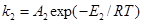(11)

where A2 is pre-exponential factor, E2 is the activation energy of the pseudo first-order acetylation reaction, R is the gas constant, and T is the absolute temperature. An Arrhenius plot of reaction rate constants, k2, is shown in Fig. 5. It is seen that the data exhibit a reasonable linear fit (R2=0.996), yielding a value of E2 of 39.6 kJ/mol, and A2 of 1.75×105 min-1.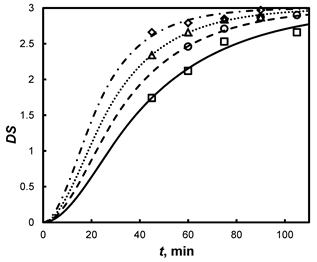Fig. 4. The measured degree of substitution, DS, as a function of the reaction time, t, at different reaction temperatures. (□) 30 °C; (○) 35 °C; (Δ) 40 °C; (◊) 45 °C; Lines: nonlinear regression by using Eqs. 8 and 10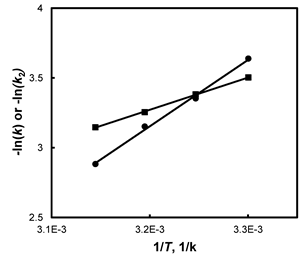Fig. 5. Arrhenius plot from the acetylation reaction rate constants, k2 (filled circles), and the dissolution rate constants, k (filled squares)

The dissolution of the sulfated chains from the surface of the solid fibers to the liquid phase can also be considered to be an activated process. Thus, the dissolution rate in Arrhenius expression may be written as,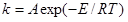(12)

where E is the activation energy of the dissolution process and is the pre-exponential factor. Therefore, the activation energy of the dissolution process may be obtained by plotting the natural logarithm of A against reciprocal absolute temperature, where, if this relationship is obeyed, a straight line will be obtained. From Fig. 5 it can be seen that the curve exhibits a good linear fit (R2=0.999), thus yielding a value of E of 19.2kJ/mol and A of 61.0 min-1.

Comparison with Literature Values

The comparison of the obtained activation energy values with those from the literature values is listed in Table 1. It can be seen that the value in this work was some 20-30 kJ/mol lower than those of similar acetylation processes, i.e. acetic acid is used as the solvents and the acetylation process is so-called ‘homogeneous process’. The discrepancy of the activation energy may be ascribed to the following two factors. One is that sulfuric acid has been examined as the better catalyst for the acetylation of activated linters than perchloric acid (Malm et al. 1961), subsequently leading to a lower activation energy in this study. The other is the different assumption of the kinetic model. For example, Frith (1963) estimated the activation energy on the assumption of pseudo first-order rate laws and obtained the value of 60.6 kJ/mol. Although Sen and Ramaswamy (1957) observed that anomalous diffusion existed in the acetylation process, they still calculated the apparent activation energy and got a value of 71.1 kJ/mol. In other words, diffusion was indeed not considered in their model.

Table 1. Literature Values for the Activation Energy for Acetylation of Cellulose with Acetic Anhydride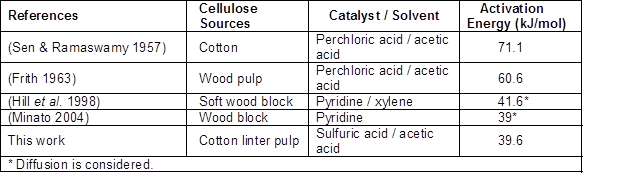The activation energy determined in this work was very close to values reported by Hill et al. (1998) and Minato (2004), although they investigated a different acetylation process; i.e. xylene was used as the solvent and the process was so-called ‘fibrous process’. As a matter of fact, Hill et al. (1998) considered the diffusion of the reagent when they estimated the activation energy; i.e. the values they got were indeed intrinsic kinetics of the acetylation of the hydroxyl groups. In the work of Minato (2004), the diffusion of the reagents was also considered and incorporated into the modified rate equation, obtaining an activation energy of 39 kJ/mol.

CONCLUSIONS

1. A kinetic model for the acetylation process of the cotton linter pulp with acetic anhydride catalyzed by sulfuric acid was proposed, in which the dissolution of the sufficiently sulfated chains on the surface of the solid fibers and the subsequent reactions in the liquid phase were considered.
2. The experiment data was regressed using a non-linear regression method. The proposed model can predict the degree of substitution (DS) of the acetylation process very well. The kinetic parameters were estimated by Arrhenius plot.
3. The chemical activation energy and the pre-exponential factor of the reactions of the cellulose hydroxyl groups with acetic anhydride were estimated as 39.6 kJ/mol and 1.75E5 1/min, respectively.
4. The dissolution rate of the sulfated chains can be represented by the equation of r/r0=1-kt. A similar equation, the Arrhenius expression, k=Aexp(-E/RT) was used to predict the dissolution rate constant. The activation energy for the dissolution process was estimated as 19.6 kJ/mol, and the pre-exponential factor was estimated as 61.0 1/min.

ACKNOWLEDGMENTS

The authors are grateful for the financial support of Nantong Cellulose Fibers Co., Ltd. We also acknowledge the financial support from the National Natural Science Foundation of China (Grant No. 21006011) and the Natural Science Foundation of Jiangsu Province (Grant No. BK2010410).

REFERENCES CITED

Araki, T. (1952). “Studies on cellulose acetate. 3. Combination of sulfuric acid during the acetylation of cellulose and the mechanism of acetylation,” Textile Research Journal 22(10), 630-636.

Dubey, A., Realff, M. J., Lee, J. H., Schork, F. J., Butte, A., Olle, B., and Kizer, L. E. (2006). “Modeling and inferential control of the batch acetylation of cellulose,” AIChE Journal 52(6), 2149-2160.

Frisoni, G., Baiardo, M., Scandola, M., Lednicka, D., Cnockaert, M. C., Mergaert, J., and Swings, J. (2001). “Natural cellulose fibers: Heterogeneous acetylation kinetics and biodegradation behavior,” Biomacromolecules 2(2), 476-482.

Frith, W. C. (1963). “Kinetics of acid catalyzed acetylation of cellulose,” Tappi 46(12), 739-744.

Goodlett, V. W., Doughert, J. T., and Patton, H. W. (1971). “Characterization of cellulose acetates by nuclear magnetic resonance,” Journal of Polymer Science Part A-1-Polymer Chemistry 9(1), 155-161.

Heinze, T., and Liebert, T. (2004). “Chemical characteristics of cellulose acetate,” Macromolecular Symposia 208, 167-237.

Hill, C. A. S., Jones, D., Strickland, G., and Cetin, N. S. (1998). “Kinetic and mechanistic aspects of the acetylation of wood with acetic anhydride,” Holzforschung 52(6), 623-629.

Malm, C. J., Tanghe, L. J., and Laird, B. C. (1946). “Preparation of cellulose acetate – action of sulfuric acid,” Industrial and Engineering Chemistry 38(1), 77-82.

Malm, C. J., Tanghe, L. J., and Schmitt, J. T. (1961). “Catalysts for acetylation of cellulose,” Industrial and Engineering Chemistry 53(5), 363-367.

Minato, K. (2004). “Application of a modified rate equation to the acetylation of wood,” Journal of Wood Science 50(5), 455-458.

Sassi, J. F., and Chanzy, H. (1995). “Ultrastructural aspects of the acetylation of cellulose,” Cellulose2(2), 111-127.

Schlufter, K., Schmauder, H. P., Dorn, S., and Heinze, T. (2006). “Efficient homogeneous chemical modification of bacterial cellulose in the ionic liquid 1-n-butyl-3-methylimidazolium chloride,” Macromolecular Rapid Communications 27(19), 1670-1676.

Sen, M. K., and Ramaswamy, M. (1957). “Kinetics of fibrous acetylation of cotton and jute,” Journal of the Textile Institute Transactions 48(3), T75-T80.

Steinmeier, H. (2004). “Chemistry of cellulose acetylation,” Macromolecular Symposia 208, 49-60.

Tang, L. G., Hon, D. N. S., and Zhu, Y. Q. (1997). “An investigation in solution acetylation of cellulose by microscopic techniques,” Journal of Applied Polymer Science 64(10), 1953-1960.

Yang, Z. P., Xu, S. W., Ma, X. L., and Wang, S. Y. (2008). “Characterization and acetylation behavior of bamboo pulp,” Wood Science and Technology 42(8), 621-632.

Article submitted: March 4, 2013; Peer review completed: April 9, 2013; Revised version received and accepted: April 15, 2013; Published: April 17, 2013.